# Define Custom TransformSelection prerequisites: Two points, one of which depends on the other

This Transform menu command defines a custom transformation.

A custom transformation is a general transformation, defined by an example that involves two points: a pre-image point and an image point that depends on the pre-image point. When you apply such a transformation to another object, every point of the object undergoes the same transformation that relates the defining points.

The relationship between the pre-image and image points can involve any combination of geometric operations (constructions and transformations) and numeric and algebraic operations (measurements, calculations, functions, and plotting). This generality makes it possible to define an extremely wide variety of custom transformations.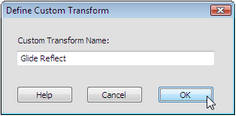To create the transformation, select the pre-image and image points and choose Transform | Define Custom Transform. In the dialog box that appears, give the transformation a name, such as Glide Reflect. This new transformation  appears as a command at the bottom of the Transform menu. To apply this transformation to another object in your sketch, select that object and choose Transform | Glide Reflect. You can also apply a custom transformations using numbered keyboard shortcuts (in Windows Ctrl+1, Ctrl+2, and so forth; on Mac,1,2, and so forth).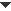Example 1: Glide Reflect

A glide reflection is a combination of two basic transformations built into Sketchpad: a reflection across a mirror line combined with a translation parallel to the mirror. To define a glide reflection as a custom transformation, reflect point A across a mirror, and then translate it by the vector from B to C, parallel to the mirror. Use points A and A'' to define the custom transformation. Then apply the transformation repeatedly to a pre-image picture to produce a glide reflection.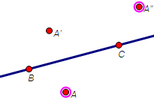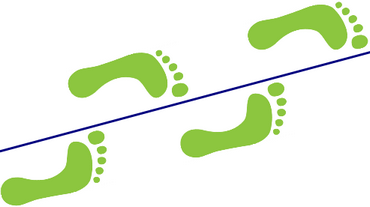Example 2: Scale Horizontally

Dilating an object scales it both horizontally and vertically. To scale an object only horizontally but not vertically, you must define a custom transformation. One way of defining such a transformation is to measure the coordinates of the point to be scaled, multiply the x-value by a scale factor (leaving the y-value unchanged), and plot the scaled point A' defined by the new coordinates. Apply the resulting transformation to a circle (to create an ellipse) or to a picture (to stretch it horizontally but not vertically).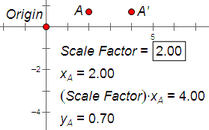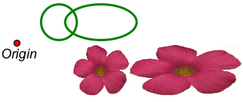Example 3: Swirl

One way to create a swirling effect is to start with a center point C and a pre-image point P and then rotate P by an angle that gets larger as P gets farther from C. To define such a rotation, measure the distance CP and calculate an angle by multiplying the distance by 10°/1 cm. (Divide by 1 cm so that the units of the result are in degrees.) Rotate P by this calculated angle and then use points P and P' to define a custom transformation. Apply the resulting custom transformation to the pre-image on the left to produce the image on the right.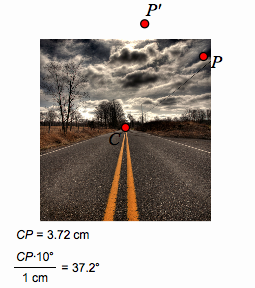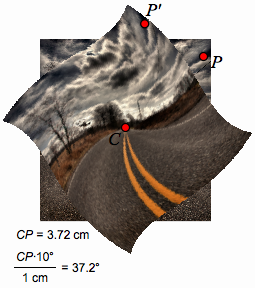Custom Transformed Images
 When a custom transformation (such as a glide reflection) is made up of one or more of Sketchpad’s basic transformations (translations, rotations, dilations, and/or reflections), images created by the custom transformation are always similar to their pre-images. Angles are the same, and distances are always in the same ratio. Transformed images of straight objects are remain straight objects, transformed images of circles remain circles, and transformed polygons are similar to their pre-image polygons. Consequently, you can use these transformed images in the same way as their pre-images: you can find the midpoint of a transformed segment, you can find the radius of a transformed circle, and you can measure the perimeter and area of a transformed polygon. But when a custom transformation (such as horizontal scaling or swirling) includes steps different from the basic transformations, there is no guarantee that the resulting images are similar to their pre-images. When a straight object (like the yellow line on the road in the example) is transformed, the result may be curved. When a circle is transformed, the result may be an ellipse, or may be some entirely different shape. The image of a polygon may not have straight edges. With such a transformation, you cannot use the transformed images in the same way as the pre-images. The image of a segment is not straight, so you cannot construct a parallel or perpendicular to it. The image of a circle isn’t circular, so you cannot measure its radius. The image of a polygon does not have straight edges, and the image of the polygon’s interior may even be outside of its frame. When a path object is transformed by such a transformation, the resulting image is a sampled transformed path such as the stretched circle in Example 2 above. Sketchpad calculates the shape of the transformed path by using points on the pre-image path and transforming them to locate the image path. Each such transformed point produces one sample on the transformed path; the full image path is made up of many such samples. Choose Edit | Properties | Plot to set the number of samples used to display such an object and to determine whether it’s displayed continuously or discretely. When a picture is transformed by such a transformation, the resulting image is a sampled transformed picture; Sketchpad calculates the transformed picture by using small rectangles from the pre-image picture and transforming them to display the resulting transformed picture. Each such transformed rectangle produces one sample of the transformed picture; the full picture is made up of many such samples. Choose Edit | Properties | Plot to set the number of samples used to display such a picture and to determine how much distortion is allowed in the sample rectangles.